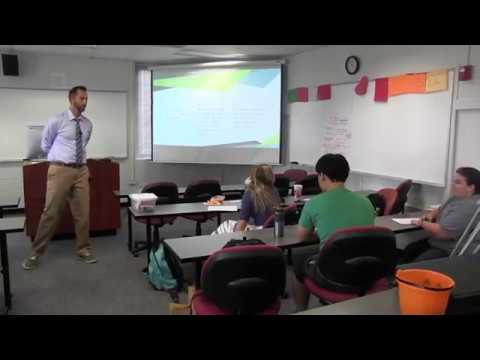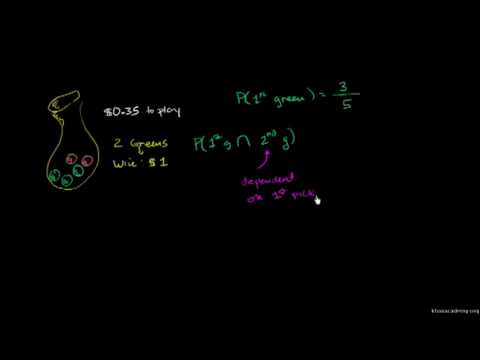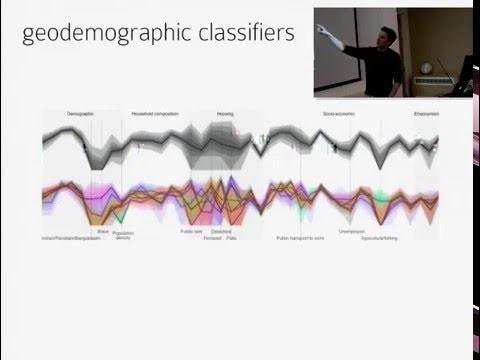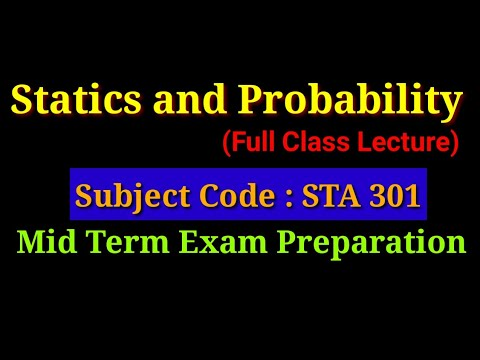Filter for:

## Showing Results For > probability

Probability for Life Science, Lecture 12, Math 3C, UCLAOriginal content provided under Creative Commons License. A math course for life science majors covering elementary probability, probability distributions, random variables, and limit theorems....

MORE
Probability for Life Science, Lecture 4, Math 3C, UCLAOriginal content provided under Creative Commons License. A math course for life science majors covering elementary probability, probability distributions, random variables, and limit theorems....

MORE
Probability Theory and Mathematical Statistics
Gina's Counters Probability TaskPractice teaching a high cognitive demand task at UGA College of Education, September 27, 2016. My first opportunity to analyze and reflect upon my questioning and instruction using a video...

MORE
Probability Part Two
Introduction to dependent probabilityThis original Khan Academy video was translated into isiXhosa by Nolusindiso Mpoyiya. The translation project was made possible by ClickMaths: www.clickmaths.org.

MORE
iGCSE Maths Nov 2007 3H Qu 8 @Monkton - Probability
SAT Maths ProbabilityProbability concepts for SAT students For private tutoring on all sections of SAT, GRE and TOEFL, contact me on silkesha@gmail.com.

MORE
VASS 2016 - Roger Beecham - Visualising Uncertainty (and pro..."Visualising Uncertainty (and probability)". A talk given by Roger Beecham at the Visual Analytics Spring School (VASS), held at Middlesex University, London, 29th March - 1st April, 2016....

MORE
Statics & Probability | Subject Code STA 301 | Full Lecture...Statics & Probability Subject Code : STA 301 Full Lecture Up2mid Exam Teacher : Sumaiya Binte Brahman Lecturer, City University, Dhaka, Bangladesh. ...

MORE
ncert class 9 maths probability exercise 15.1 solution to qu...ncert class 9 maths probability exercise 15.1 solution to question 12,13.

MORE
Probability for Life Science, Lecture 5, Math 3C, UCLAOriginal content provided under Creative Commons License. A math course for life science majors covering elementary probability, probability distributions, random variables, and limit theorems....

MORE
Computer-science:   |  graphics  |  website building  |  algorithms  |  javascript  |  java  |  phython  |  sql databases
•Share on
Facebook

•Share on
Twitter

•Share on
Google+

•Share on
LinkedIn

•Share by
Mail